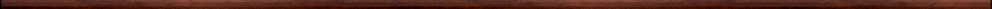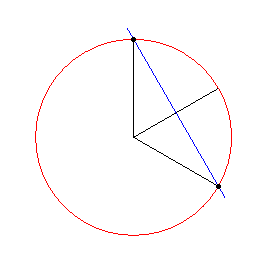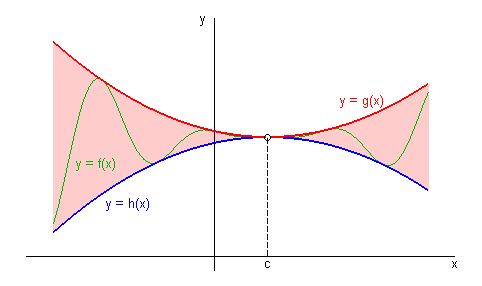Maple worksheets giving an introduction to calculus, limits and continuity.Basic calculus topics:

They are all compatible with Classic Worksheet Maple 10.Introduction - intro.mws

• Introduction .. the basic problems of differential and integral calculus.
• The equations and gradients of tangent lines.

A procedure for constructing secant to tangent animations - tgt_anim.mws

• A procedure which gives the line through two points: linethrough.
• Secant lines.
• A procedure for constructing secant to tangent animations: secant_to_tangent.
• Tangent lines.
• A procedure for finding and plotting tangent lines: findtangent.

Limits - limmits.mws

• The concept of the limit of a function and limit notation.
• First non-trivial example of the limit of a function: (x^3-8)/(x-2) -> 12 as x -> 2.
• Finding limits algebraically.
• Using the Maple procedure: limit.
• Graphical illustration of limits.

Evaluating limits using basic properties of limits - limits2.mws

• Properties of limits.
• Replacing the function in a limit by a function that is continuous at the limiting point.
• The Squeeze Theorem for limits.
• Two special trigonometric limits.

Infinite  limits - limits3.mws

• Intoductory example and definitions involving one-sided limits.
• Vertical asymptotes.

A procedure for evaluating limits - limits4.mws

• A procedure for evaluating limits that shows the working for "evaluation by hand": eval_lim/Limit.
• Examples.

Using the procedure eval_lim/Limit to evaluate limits from a typical calculus text - limits5.mws

• Examples.

Continuous and discontinuous functions - contfcns.mws

• Introductory example of a discontinuous function
• More examples of discontinuous functions.
• Breaking down checking of continuity of a function at a point into three steps.

More on continuity of functions - contfcns2.mws

• Removable and non-removable discontinuities.
• Continuity on general intervals and classes of continuous functions.
• The Intermediate value Theorem.

Calculus procedures - calculus.zipTop of page

Main index